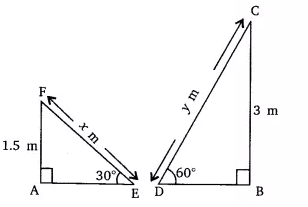Q

# A contractor plans to install two slides for the children to play in a park. For the children below the age of 5 years, she prefers to have a slide whose top is at a height of 1.5 m, and is inclined at an angle of 30° to the ground, whereas for elder

3. A contractor plans to install two slides for the children to play in a park. For the children below the age of 5 years, she prefers to have a slide whose top is at a height of 1.5 m, and is inclined at an angle of 30° to the ground, whereas for elder children, she wants to have a steep slide at a height of 3m, and inclined at an angle of 60° to the ground. What should be the length of the slide in each case?

ViewsSuppose  m is the length of slides for children below 5 years and the length of slides for elders children be  m.

Given that,
AF = 1.5 m, BC = 3 m,  and

In triangle EAF,

The value of  is 3 m.

Similarily in CDB,

the value of  is  = 2(1.732) = 3.468

Hence the length of the slide for children below 5 yrs. is 3 m and for the elder children is 3.468 m.

Exams
Articles
Questions